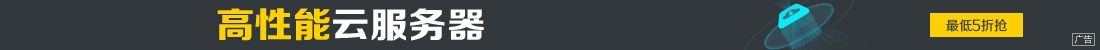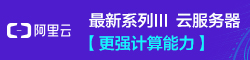# java 数据结构与算法 (快速排序法)

••p 元素的值 就是 2对应的索引 0 加上 5对应的索引 7 之和 除以2 得到 索引为3 对应的元素7```import java.util.Arrays;

public class kuaisu {
public static void main(String[] args) {
int arrays[]=new int[]{2,9,4,7,3,3,6,5};
sort(arrays,0,arrays.length-1);
System.out.println(Arrays.toString(arrays));

}```
```  public  static  void  sort(int arrays[],int left,int right){
int l=left ;//给定下标
int r=right;//给定下标
int temp; //定义一个变量 作为中间值 交换 左右两边的元素位置
int pivot=arrays[(left+right)/2];//中间值
while(l<r){
//在左边查找小于中间值得元素
while(arrays[l]<arrays[pivot]){
l++;

}
//同理在右边查找大于中间值得元素
while(arrays[r]>arrays[pivot]){
r--;
}//直到左边元素大于右边元素就结束
if(l>=r){
break;
}
temp=arrays[l];
arrays[l]=arrays[r];
arrays[r]=temp;
//交换完arrays[l]=pivot
if(arrays[l]==pivot){
r--;
}
if(arrays[r]==pivot){
l++;
}
if(r==l){ //要让左边元素 往左边移 右边元素往右边移 错开
l++;
r--;
} //对左边进行递归
if(left<r){
sort(arrays,left,r);
}//对右边进行递归
if(right>l){
sort(arrays,l,right);

}
}
}
}```